Home

# CONVOLUTION OF ORBITAL MEASURES ON SYMMETRIC SPACES OF TYPE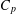$C_{p}$ AND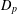$D_{p}$

## Abstract

We study the absolute continuity of the convolution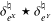${\it\delta}_{e^{X}}^{\natural }\star {\it\delta}_{e^{Y}}^{\natural }$ of two orbital measures on the symmetric spaces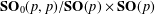$\mathbf{SO}_{0}(p,p)/\mathbf{SO}(p)\times \mathbf{SO}(p)$ ,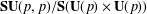$\mathbf{SU}(p,p)/\mathbf{S}(\mathbf{U}(p)\times \mathbf{U}(p))$ and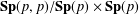$\mathbf{Sp}(p,p)/\mathbf{Sp}(p)\times \mathbf{Sp}(p)$ . We prove sharp conditions on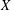$X$ ,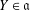$Y\in \mathfrak{a}$ for the existence of the density of the convolution measure. This measure intervenes in the product formula for the spherical functions.

• # Send article to Kindle

Note you can select to send to either the @free.kindle.com or @kindle.com variations. ‘@free.kindle.com’ emails are free but can only be sent to your device when it is connected to wi-fi. ‘@kindle.com’ emails can be delivered even when you are not connected to wi-fi, but note that service fees apply.

Find out more about the Kindle Personal Document Service.

CONVOLUTION OF ORBITAL MEASURES ON SYMMETRIC SPACES OF TYPE$C_{p}$AND$D_{p}$
Available formats
×

# Send article to Dropbox

To send this article to your Dropbox account, please select one or more formats and confirm that you agree to abide by our usage policies. If this is the first time you use this feature, you will be asked to authorise Cambridge Core to connect with your <service> account. Find out more about sending content to Dropbox.

CONVOLUTION OF ORBITAL MEASURES ON SYMMETRIC SPACES OF TYPE$C_{p}$AND$D_{p}$
Available formats
×

# Send article to Google Drive

To send this article to your Google Drive account, please select one or more formats and confirm that you agree to abide by our usage policies. If this is the first time you use this feature, you will be asked to authorise Cambridge Core to connect with your <service> account. Find out more about sending content to Google Drive.

CONVOLUTION OF ORBITAL MEASURES ON SYMMETRIC SPACES OF TYPE$C_{p}$AND$D_{p}$
Available formats
×

## References

Hide All
MathJax
MathJax is a JavaScript display engine for mathematics. For more information see http://www.mathjax.org.

# CONVOLUTION OF ORBITAL MEASURES ON SYMMETRIC SPACES OF TYPE$C_{p}$ AND$D_{p}$

## Metrics

### Full text viewsFull text views reflects the number of PDF downloads, PDFs sent to Google Drive, Dropbox and Kindle and HTML full text views.

Total number of HTML views: 0
Total number of PDF views: 0 *Loading metrics...

### Abstract viewsAbstract views reflect the number of visits to the article landing page.

Total abstract views: 0 *Loading metrics...

* Views captured on Cambridge Core between <date>. This data will be updated every 24 hours.

Usage data cannot currently be displayed.